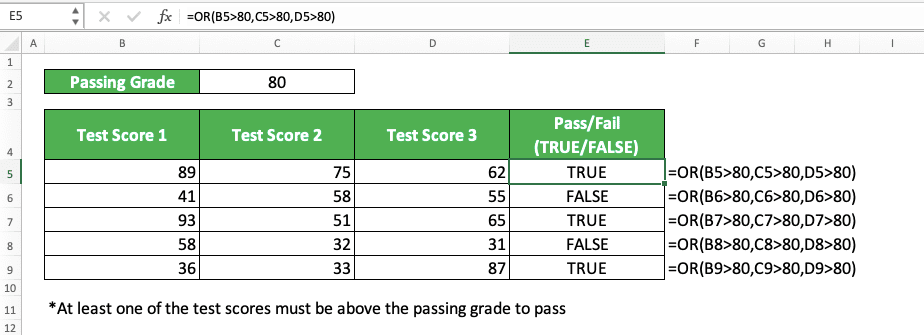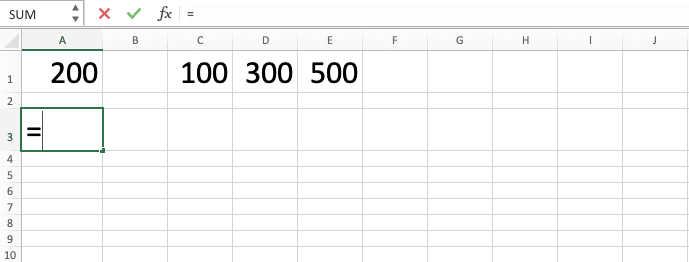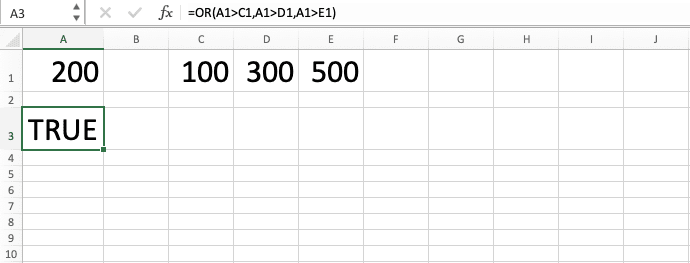OR Excel Formula: Functions, Examples, and How to Use - Compute Expert

# OR Excel Formula: Functions, Examples, and How to Use

Home >> Excel Tutorials from Compute Expert >> Excel Formulas List >> OR Excel Formula: Functions, Examples, and How to Use

In this tutorial, you will learn completely about the usage of the OR excel formula.

In excel, we sometimes need to get a combined logic value from multiple logic conditions. OR can help us to do that as long as its unique combination process is what we actually need.

Curious to know more about the usage of this excel formula? Learn more from this OR tutorial!

Disclaimer: This post may contain affiliate links from which we earn commission from qualifying purchases/actions at no additional cost for you. Learn more

## OR Function in Excel

We can use OR to get a combined logic value from multiple logic conditions. OR will produce TRUE if at least one of them is TRUE and FALSE if all of them are FALSE.

## OR Result

The result we get from OR is a TRUE or FALSE logic value depending on the logic conditions we input.

## Excel Version from Which We can Start to Use OR

We can start using the OR formula in excel since excel 2003.

## The Way to Write It and Its Inputs

Generally, you can write OR in excel like this.

= OR ( logic_condition1 , [ logic_condition2 ] , … )

The inputs of OR are all the logic conditions you want to combine to get your combined logic value. You can input up to 255 logic conditions in OR.

You can also input a logic value (TRUE or FALSE) directly although it is rare that we should do that. OR will produce TRUE if at least one of its inputs has a TRUE value. Otherwise, it will produce FALSE if all of them are FALSE.

## Example of Its Usage and Result

The following is the implementation and result example of OR in excel.In the example, we want to get the pass/fail (TRUE/FALSE) result of each test score row. The passing requirement is that at least one of the three test scores must pass the minimum score (80).

For this condition, it will be ideal if we use OR to get the pass/fail result.

OR will produce TRUE if at least one of its logic condition inputs is TRUE and FALSE if all are FALSE. For the example, we input all the test score conditions that must be above 80. We write something like“test_score>80” for each of the logic conditions.

We input all of the test scores per row in our OR formula writing. As a result, we get the pass/fail results in the form of TRUE/FALSE logic values!

## Writing Steps

After we have discussed the OR writing form, inputs, and example, now let’s discuss its writing steps. If you have learned the tutorial until this point, then you should master the implementation of these steps quite easily!

1. Type an equal sign ( = ) in the cell where you want to put the OR result in2. Type OR (can be with large and small letters) and an open bracket sign after =3. Input all the logic conditions that you want to get your combined logic value from. Type comma signs ( , ) between each of the logic condition inputs4. Type a close bracket sign when all of your logic conditions have been inputted5. Press Enter
6. The process is done!## OR for IF with Multiple Logic Conditions: IF OR Excel Statement

As OR produces a TRUE/FALSE logic value, we usually use it as an input to our IF formula. This is because IF needs input like that and we seldom need logic values as final results in excel.

As we can only input one logic condition in a normal IF, OR can help us to input more than one. We can also use AND for this although it has a different process compared to OR (AND will produce TRUE if all of its logic condition inputs are TRUE. It will produce FALSE otherwise).

To make OR as an input in IF, here is the formula combination general writing form.

= IF ( OR ( logic_condition1 , [ logic_condition2 ] , … ) , result_if_true , result_if_false )

In the IF OR combination, we input our OR in the first input part of the IF. That part is the IF logic condition input part.

We input all the logic conditions that we want to combine for our IF in our OR. From the OR, we will get a TRUE or FALSE logic value. This logic value will determine whether we will get the TRUE or FALSE result from our IF.

To better understand the concept, here is an example of an IF OR implementation in excel.Here, we use the same data as we use for the OR formula example earlier. However, now we want to get a pass/fail mark for each test score row, not just a TRUE/FALSE.

For that, we need to use IF with OR. As previously explained, to do the combination, we type OR as the logic condition input in our IF.

We input all the test score conditions in each row to pass (test_score>80) to our OR. After that, we input the IF result if the OR produces TRUE and if it produces FALSE. In the example, that means we put the “pass” mark for TRUE and we put the “fail” mark for FALSE.

We write our IF OR formulas like that and the result is as you can see in the example screenshot! We get all the pass/fail results correctly from those IF OR that we write.

## Exercise

After you learned how to use the OR formula in excel, now please practice your understanding by doing the exercise below!

### Questions

In a school where the scholarship requirements is very tight...
1. A student will pass test group 1 if his/her score is >=90 in at least one test. Check whether each student passes test group 1 or not and write the result in the provided column! Use TRUE or FALSE as the content of each column cell to give the answer!
2. A student will pass test group 2 if his/her score is >=95 in at least one test. Check whether each student passes test group 2 or not and write the result in the provided column! Use TRUE or FALSE as the content of each column cell to give the answer!
3. A student will get a scholarship if he/she pass one of the test group. Check whether each student gets the scholarship or not and write the result on the provided column! Use “Scholarship” or “No Scholarship” as the content of each column cell to give the answer!

• OR isn’t case sensitive in its processing
• If we just input text or blank cells to OR, it will ignore them

Other tutorials you should learn from:

Get updated excel info from Compute Expert by registering your email. It's free!